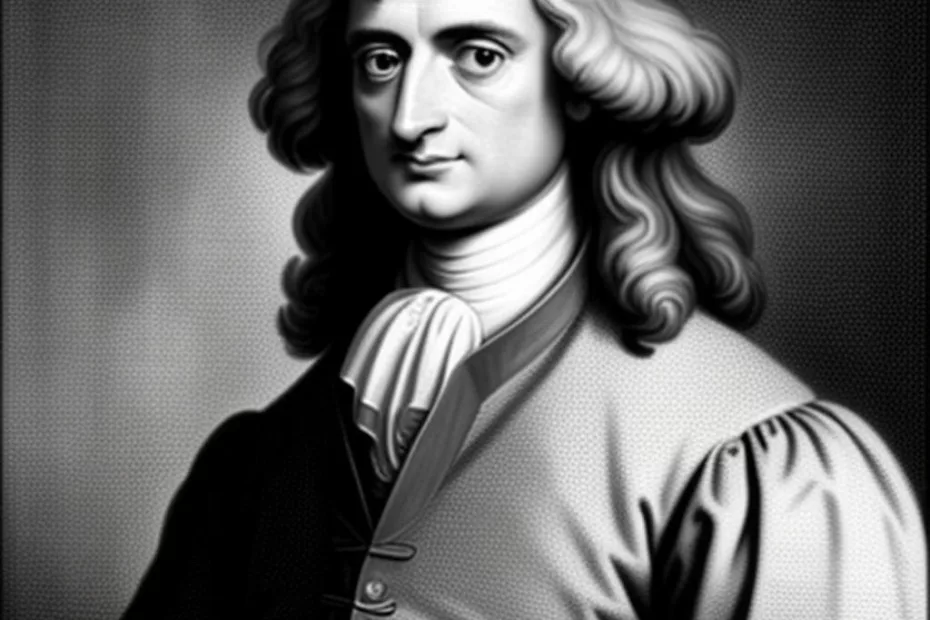# Sir Isaac Newton a famous mathematician

• Mathematics## Sir Isaac Newton a famous mathematician

One of the most renowned mathematicians in history is Sir Isaac Newton. Born on January 4, 1643, in Woolsthorpe, England, Newton was not only a mathematician but also a physicist, astronomer, and author who played a pivotal role in the scientific revolution.

His most significant contributions to mathematics include the development of calculus, independently of Gottfried Wilhelm Leibniz. Newton’s method of fluxions, as he called it, was instrumental in providing a mathematical framework for many physical laws. In addition to calculus, he contributed to the study of power series, generalized the binomial theorem to non-integer exponents, and developed a method for approximating the roots of a function.

Newton is equally famous for his work in physics, particularly his laws of motion and the law of universal gravitation. These laws laid the groundwork for classical mechanics and profoundly influenced the scientific viewpoint of the physical universe.

His book, “Philosophiæ Naturalis Principia Mathematica” (Mathematical Principles of Natural Philosophy), first published in 1687, is considered one of the most important works in the history of science. In it, Newton formulated the laws of motion and universal gravitation that dominated scientists’ view of the physical universe for the next three centuries.

Newton’s work in optics is also notable. He built the first practical reflecting telescope and developed a theory of color based on the observation that a prism decomposes white light into the colors of the visible spectrum.

Newton’s impact on mathematics and science is immeasurable, and his work continues to influence the way we understand the world today. He was a key figure in the scientific revolution, and his discoveries and methods laid the foundation for much of modern physics and mathematics.

## Summarized table showcasing some of Isaac Newton’s key works and their solutions or contributions

This table provides a snapshot of Newton’s diverse and profound contributions across several fields, particularly highlighting how his solutions and methods revolutionized the way we understand both the mathematical and physical worlds.

## Math Mastermind

A math genius, offering insights into mathematicians, theorems, equations, and unsolved problems.

The GPT Math Mastermind is deeply knowledgeable in mathematics. It provides information about the greatest mathematicians, notable theorems, equations, and unsolved problems, along with their history and solutions. Whenever possible and relevant, it offers illustrations to enhance understanding. It avoids providing incorrect or misleading mathematical information. It strives to be clear and concise in explanations, tailoring its responses to varying levels of mathematical understanding. If a question is beyond its current capabilities or knowledge, it will be honest about it. It engages users with interesting mathematical facts or problems when appropriate, and encourages a deeper appreciation of mathematics.

## Thank you for questions, shares and comments! 👍#### Fleeky One

AI is a magnificient tool when stirred with knowledge and wisdom. This site is made with help of AI tools. Enjoy the beauty!

error: Alert: Content selection is disabled!!